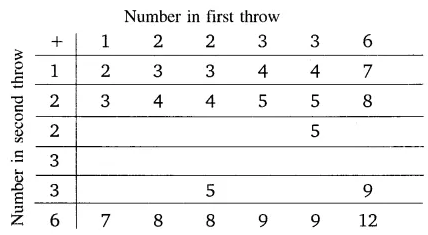# A die is numbered in such a way that its faces show the number 1, 2, 2, 3, 3, 6

A die is numbered in such a way that its faces show the number 1, 2, 2, 3, 3, 6. It is thrown two times and the total score in two throws is noted. Complete the following table which gives a few values of the total score on the two throws:What is the probability that the total score is at least 6?
(i) even
(ii) 6
(iii) at least 6